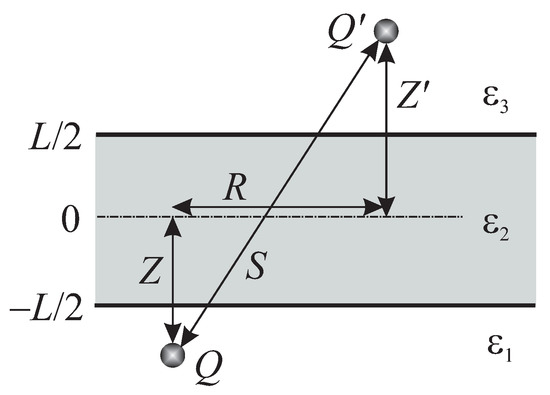Next Article in Journal
High Precision Test of the Pauli Exclusion Principle for Electrons
Previous Article in Journal
Superconducting Properties of 3D Low-Density TI-Bipolaron Gas in Magnetic Field
Open AccessArticle

# Electrostatic Interaction of Point Charges in Three-Layer Structures: The Classical Model

Institute of Physics, 46 Nauka Ave., 03680 Kyiv, Ukraine
*
Author to whom correspondence should be addressed.
Condens. Matter 2019, 4(2), 44; https://doi.org/10.3390/condmat4020044
Received: 4 April 2019 / Revised: 27 April 2019 / Accepted: 29 April 2019 / Published: 2 May 2019
Electrostatic interaction energy W between two point charges in a three-layer plane system was calculated on the basis of the Green’s function method in the classical model of constant dielectric permittivities for all media involved. A regular method for the calculation of $W ( Z , Z ′ , R )$ , where Z and $Z ′$ are the charge coordinates normal to the interfaces, and R the lateral (along the interfaces) distance between the charges, was proposed. The method consists in substituting the evaluation of integrals of rapidly oscillating functions over the semi-infinite interval by constructing an analytical series of inverse radical functions to a required accuracy. Simple finite-term analytical approximations of the dependence $W ( Z , Z ′ , R )$ were proposed. Two especially important particular cases of charge configurations were analyzed in more detail: (i) both charges are in the same medium and $Z = Z ′$ ; and (ii) the charges are located at different interfaces across the slab. It was demonstrated that the W dependence on the charge–charge distance $S = R 2 + Z − Z ′ 2$ differs from the classical Coulombic one $W ∼ S − 1$ . This phenomenon occurs due to the appearance of polarization charges at both interfaces, which ascribes a many-body character to the problem from the outset. The results obtained testify, in particular, that the electron–hole interaction in heterostructures leading to the exciton formation is different in the intra-slab and across-slab charge configurations, which is usually overlooked in specific calculations related to the subject concerned. Our consideration clearly demonstrates the origin, the character, and the consequences of the actual difference. The often used Rytova–Keldysh approximation was analyzed. The cause of its relative success was explained, and the applicability limits were determined. View Full-Text
Show FiguresFigure 1

MDPI and ACS Style

Gabovich, A.M.; Voitenko, A.I. Electrostatic Interaction of Point Charges in Three-Layer Structures: The Classical Model. Condens. Matter 2019, 4, 44.